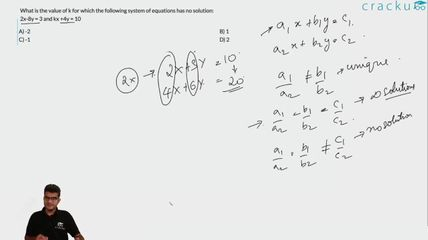Question 13

# What is the value of k for which the following system of equations has no solution: 2x-8y = 3 and kx +4y = 10

Solution

On solving both equations, we will get $$x = \frac{23}{2+2k}$$
now for having no solutions to system 2+2k should be 0.
Hence k=-1

### View Video Solution